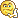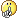#www.vustudents.ning.com

We non-commercial site working hard since 2009 to facilitate learning Read More. We can't keep up without your support. Donate.

CS201 All Current Final Term Papers Fall 2012 (20 February to 03 March 2013) at one Place

From 20 February to 03 March 2013 Fall 2012

Current Final Term Papers Fall 2012 Papers, Feb 2013 Final Term Papers, Solved Final Term Papers, Solved Papers, Solved Past Papers, Solved MCQs

Please Share your Current Papers Questions/Pattern here to help each other. Thanks

Views: 5658

### Replies to This Discussion

cs201-today paper few questoins

These are four questions, 5 marks each.

Today’s CS201 paper was mainly from lectures 10-40. A greater portion was from 15-35. Most of the questions were from pointers, arrays and dynamic memory allocation. Four questions of 5 marks each were,

1-      Write statement for constant pointer to integer and also pointer to constant integer.

2-      Get input from user for 2 by 4 matrix and display it.

3-      For given code of class rectangle, write code for copying constructor.

4-      How will you identify if a declared variable if reference of address variable.

Some other questions were,

For given code having new operator, write delete.

For given code for converting Celsius to Fahrenheit, write equivalent template.

today was my paper of cs201.

ishtiaq mehmood Share your paper questionsMy today cs201 final term paper

02-03-2013

MCQ’s new thy sab koi 4,5 past paper mein sy thy subjective paper ye tha

Q: 1        In C++ language how would you identify whether a declared variable is a “reference variable “ or an “address variable” ?

Q: 2        How can we initialize an object which is declared as constant data member in another class ?

Q:3         write general syntax of two way friendship between the two classes (class1  and class2) ?

Q:4         Assume if p is a pointer then what will happen if  each of the below statement is executed ?

• Delete  (p)
• Delete []p

Q:5         what is the difference between “endl” and “ \n” ?

Q:6         write the output of the program

#include <iostream.h>

Main ( )

{

Char *name [] = {“Ali” , “Ahmad” , “Riaz”};

int x;

int y;

x = sizeof (name);

y = sizeof (*name);

cout x endl y ;

return 0 ;

}

Q:7         write the output of the following code

#include < string.h >

#include < iostream.h >

int main ( )

{

Char str [ ] = “ This is a line “ ;

Char*p ;

P = strchr ( str , ‘s’ ) ;

While ( p! = NULL ) {

Cout “ found at \n “ p – str + 1 ;

P = strchr ( p + , ‘s’ ) ;

return 0 ;

}

Q:8         write a program which defines a user-defined manipulator named Octal. This manipulator should convert and display the decimal number into Octal form. In the main function, you have to take a decimal number as an input from the user and hen by using the user defined manipulator display the number in Octal form.

Q:9         write a program that defines a Template function named Square ( ) which finds the square of a number and then return it. Define one variable of type float in main function and call square function on that.

Output should be as given below

Enter a float value to its square: 12.3

Square of float number is: 151.29

3 question yaad nai aa rhy

myra aj jo paper hwa us main jis kism k questions thy wo in topics per thy:

2. Two questions were about nested Classes and aik main program member access code bnana tha and 2nd kuch youn tha

" If class A, B and C are three classes with class A has Class B as friend Class and Class B has C as friend Class..then explain that do there exist the associativity and Transitivity in these classes.

3. Copy cunstructor  and destructork bary main 8 to 10 marks tk k questions thy (3+ 2+ 2+1) tak

4.pointers ko use kr k array elements ko reverse print krwana tha 5 marks

5.Objective ziada tar Operators, Classes, memory allocation , constructor, destructor, 5end chapters sy tha.........

Aoa ,Plz  any one tell me about    ,k maaz ki file (MCQ+subjective)sy kuch aya hy final exam may ,k nai ?CS201 some current Final Term Papers 2013

February 20, 2013
1)Write a simple program, which will get a stream of character and output the stream of characters using cin and cout.
2) Find the error in following syntax,
while(i<=100)
sum+=i*i;
. class String
{
char buf ;
};
Write code for assignment (=) operator function which assign one String object to other object. Your code should also avoid self assignment.
3).make a program using template function select two floating point number and add them with add() function.(
n February 20, 2013 at 4:00pm
there are some current questions .
Write a program which consists of two classes, Date and Person. Date class should contain three data members day , month , year and setter and getter function for these data members. Date class should also contain showdate() member function to display date.
Person class should contain three data members Name, Address, and Bday , where Name and Address are char pointer while Bday(Date of birth) is of type Date, Person class should further contain two member functions Display() and setdate().
In main program Create an object of Class person and call the member functions with it..
February 20, 2013 at 4:28pm
MY CS201 Current Paper:
20-2-2013
Total 52 Qs
40 mcqs …..only 20% were from past papers:
Subjective Qs were almost 90% from past papers … Some Qs which I remember
1) What happens when we use new and delete operator? (from past papers)
2) Question: Suppose an object of class A is declared as data member of class B.
(i) The constructor of which class will be called first? Answer A
(ii) The destructor of which class will be called first? Answer B(from past papers)
3) Write a program which defines five variables which store the salaries of five employees, using setw and setfill manipulators to display all these salaries in a column. Note: Display all data with in a particular width and the empty space should be filled with character x Output should be displayed as given below: (from past papers)
xxxxxx1000
xxxxxx1500
xxxxx20000
xxxxx30000
xxxxx60000
4)Overflow condition is used in repetition structure at which point it oocurs?
5)Is it possible to overlad operation for primitive data types.justify yor ans
6) Let we have a class,
class String
{
private: char buf;
}; Write code for assignment (=) operator function which assign one String object to other object. Your code should also avoid self assignment(from past paper)
7)What happen when an object is assigned to another object without overloading an assignment operator?
8)Write function name sum which accepts an integer as a argument in the function use a static variable to add the value passed to it as argument with each function call is the endl display the sum of value pass an argument:
9) Question No: 40 ( Marks: 10 ) Write a program which consists of two classes, Date and Person. Date class should contain three data members day , month , year and setter and getter function for these data members. Date class should also contain showdate() member function to display date.
Person class should contain three data members Name, Address, and Bday , where Name and Address are char pointer while Bday(Date of birth) is of type Date, Person class should further contain two member functions Display() and setdate().
In main program Create an object of Class person and call the member functions with it..(from Past Papers.

Today Final Term Paper Fall 2012
On 21 Feb 2013
Total Questions: 52
Total Marks: 80
Total MCQs: 40 (Each of 1 Mark)
Total Short Questions: 4 (Each of 2 Mark)
Total Short Questions: 4 (Each of 3 Mark)
Total Long Questions: 4 (Each of 5 Mark)
CS201

Q1: (Marks 5) Write a program that defines a Template function named Add () which adds two numbers returns Sum. Define two variables of int type in the main and then call Add () function on that.
Output should be like this:

Adding numbers (don’t remember here what was written)
Enter first number:
12
Enter second number:
25
Sum=37

Q2: (Marks 5) Find the error in the program and then remove this. (Don’t remember the coding)

Q3: (Marks 5) Given the class Data

main()
{
class Date
{
private:
int day, month, year;
public:
day=01;
month=10;
year=2010
}
……………..Dont remember the lines
……………..
……………..

}

Use the dynamically allocation (don’t remember what)
In the last also free memory allocation.

Q4: (Marks 3) Why goto is not recommended to use in programmes?
Q5: (Marks 2) Write two types of data conversions used for used defined data types.
Q6: (Marks 2) Write the following using logical operators:
1. number is both multiple of 4 and 6
2. number is even but not 8
3. answer is either N or n
4. don’t remember this

Q7: (Marks 3) Write the output of the following if “int 5” is passed to the variable:
Template <class T);
T reciprocal (T x);
{
return (1/x);
}
(it was like something. Don’t remember exactly)

Q8: (Marks 3): How can we determine that dynamically memory allocation has been set successfully or not is C++ using the new operator?
February 21, 2013 at 6:37pm
Total Questions: 52
Total Marks: 80
Total MCQs: 40 (Each of 1 Mark)
Total Short Questions: 4 (Each of 2 Mark)
Total Short Questions: 4 (Each of 3 Mark)
Total Long Questions: 4 (Each of 5 Mark)

Q.make a program using getline function
Q.make a program using template function select two floating point number and add them with add() function.(5)
Q.make a program take salary and pay tax of 5% if salary is less than 10,000 and pay 7% if the salary is greater than 10,000 using switch function.
Q.what are the function of new and delete ?
Q.what are the stream insertion overloaded operators?
Q Write a program which open a file name “this.txt” and then using write() function, writes the string “Introduction to Programming” at the end of the file.
Q What will be the output of the program:
Int x=10;
Int&y=x;
Y+=5;
Coutx;
Q A two dimensional array has 3 rows and 4 columns. Write down the syntax to initialize first element of all three rows of two dimensional array with value 2.
Q How can we initialize an object which is created as constant data member in another class?
Q An array delare int day[]={1,2,3,4,5,6,7}
How many elements have?
If the declaration ofarray is changed to int days={1,2,3,4,5,6,7}
How many elements have?